## Tuesday, August 25, 2009

### Discrete Maths, ProblemSet#3

These are my solutions to 3rd problem set of discrete mathematics course taught at Arsdigita university by Shai Simonson. Other posts relating to this are here.

The code for various problems is here...
`;P-2c(define nil '());this is a LIFO stack(peg for TOH) in which you can put;numbers(number represents disk);bigger number represents a disk with bigger size;Each peg/stack has a name also(define (make-disk-stack stack-name)  (let ((disks nil))    (define (put-disk n)      (if (and (not (null? disks)) (> n (car disks)))          (error "ERROR: trying to put bigger disk             over smaller disk. new-disk: " n              " on stack:" stack-name disks)          (set! disks (cons n disks))))    (define (remove-disk)      (if (null? disks)          (error "Error: No disk is available." stack-name)          (let ((tmp (car disks)))            (set! disks (cdr disks))            tmp)))    (define (size)      (length disks))    (define (print)      (display disks)      (newline))    (define (name)      stack-name)    (define (dispatch msg . args)      (cond       ((eq? msg 'put-disk) (put-disk (car args)))       ((eq? msg 'remove-disk) (remove-disk))       ((eq? msg 'size) (size))       ((eq? msg 'print) (print))       ((eq? msg 'name) (name))       (else (error "Error: msg not recognized." stack-name))))    dispatch))(define (put-disk n stack)  (stack 'put-disk n))(define (remove-disk stack)  (stack 'remove-disk))(define (size stack)  (stack 'size))(define (print stack)  (stack 'print))(define (name stack)  (stack 'name));create stack containing n disks(define (fill-stack stack n)  (if (= n 0) stack      (begin (put-disk n stack)             (fill-stack stack (- n 1)))));=====================================;just to check that my approach is correct, I'm doing;the standard TOH algo here(define (Standard-TOH from to using n)  (if (= n 1)      (begin        (display (string-append "moving disk from " (name from)                                 " to " (name to)))        (newline)        (put-disk (remove-disk from) to))      (begin        (Standard-TOH from using to (- n 1))        (put-disk (remove-disk from) to)        (Standard-TOH using to from (- n 1)))));lets do for 7 disks(define to (make-disk-stack "TO"))(define from (fill-stack (make-disk-stack "FROM") 7))(Standard-TOH from to (make-disk-stack "USING") 7)(print to) ;should print (1 2 3 4 5 6 7)(print from) ;should print ();==================================;Sloppy Joe's algorithm       (define (TOH from to using1 using2 n)  (if (= n 1)      (begin        (display (string-append                   "moving disk from " (name from) " to " (name to)))        (newline)        (put-disk (remove-disk from) to))      (begin        (TOH from using1 to using2 (/ n 2))        (TOH from to using1 using2 (/ n 2))        (TOH using1 to from using2 (/ n 2)))))(TOH (fill-stack (make-disk-stack "FROM") 4)      (make-disk-stack "TO")      (make-disk-stack "USING1") (make-disk-stack "USING2") 4);ERROR: trying to put bigger disk over ;smaller disk. new-disk:  3 " stack:" (1 2)(TOH (fill-stack (make-disk-stack "FROM") 8)      (make-disk-stack "TO")      (make-disk-stack "USING1") (make-disk-stack "USING2") 8);ERROR: trying to put bigger disk over ;smaller disk. new-disk:  3 " stack:" (1 2);Fruity Freddie algorithm       (define (TOH from to using1 using2 n)  (if (= n 1)      (begin        (display (string-append                   "moving disk from " (name from) " to " (name to)))        (newline)        (put-disk (remove-disk from) to))      (begin        (TOH from using1 to using2 (/ n 2))        (TOH from to using2 using1 (/ n 2))        (TOH using1 to from using2 (/ n 2)))))(TOH (fill-stack (make-disk-stack "FROM") 4)      (make-disk-stack "TO")      (make-disk-stack "USING1") (make-disk-stack "USING2") 4); Finishes successfully(TOH (fill-stack (make-disk-stack "FROM") 8)      (make-disk-stack "TO")      (make-disk-stack "USING1") (make-disk-stack "USING2") 8);ERROR: trying to put bigger disk over ;smaller disk. new-disk:  7 " stack:" (1 2 3 4);3 a,b;steps;if n = 1 then move 1 disk from From to To;else;Move n/2 disks to Using1 from From, using To and Using2;Move remaining n/2 disks from From to To using Using2 only with;standard TOH algorithm;Move n/2 disks from Using1 to To using From and Using2(define (TOH from to using1 using2 n)  (if (= n 1)      (begin        (display (string-append                   "moving disk from " (name from) " to " (name to)))        (newline)        (put-disk (remove-disk from) to))      (begin        (TOH from using1 to using2 (quotient n 2))        (Standard-TOH from to using2 (- n (quotient n 2)))        (TOH using1 to from using2 (quotient n 2)))));lets do for 16 disks(define to (make-disk-stack "TO"))(define from (fill-stack (make-disk-stack "FROM") 15))(TOH from to (make-disk-stack "USING1") (make-disk-stack "USING2") 15)(print to) ;should print (1 2 3 4 5 6 7 8 9 10 11 12 13 14 15)(print from) ;should print ();11a;Algorithm;num = (d1 d2 ...dn);result = (g1 g2 ...gn);start with dn, if dn-1 = 1 then gn=1-dn or gn=dn;go all the way till d1, d0 is assumed to be 0;so g1 = d1(define (bin->gray num)  (define (iter n a)    (if (null? (cdr n))        (cons (car n) a)        (if (= (cadr n) 1)            (iter (cdr n) (cons (- 1 (car n)) a))            (iter (cdr n) (cons (car n) a)))))  (iter (reverse num) nil));11b(define (gray->bin num)  (define (iter n a)    (if (null? n) a        (if         (= (modulo (reduce + 0 (cdr n)) 2) 1)         (iter (cdr n) (cons (- 1 (car n)) a))         (iter (cdr n) (cons (car n) a)))))  (iter (reverse num) nil))`

Here are my solutions...

### Discrete Maths, Lecture-10

These are my notes from 10th lecture of discrete mathematics course taught at Arsdigita university by Shai Simonson. Other posts relating to this are here.

This lecture covers basic induction with Josephus problem as an example. Here are my notes...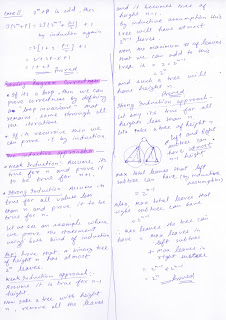## Monday, August 24, 2009

### Discrete Maths, Lecture-8,9 [Solving Recurrance relations]

These are my notes from 8th and 9th lecture of discrete mathematics course taught at Arsdigita university by Shai Simonson. Other posts relating to this are here.

These lectures cover the methods of solving recurrence relations, which are...
• Repeated Substitution
• Master Method
• Change of Variables
• Guess and prove by induction
• Linear homogeneous/non-homogeneous recurrence solving methods
Here are my notes...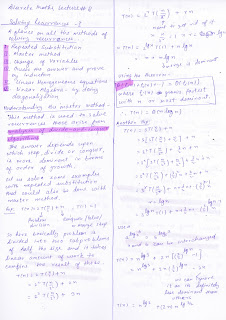## Sunday, August 23, 2009

### covariance-contravariance and constraining type params

If you define a class saying something like "class Test[A]" in scala then..
• It is important to notice that Test is not a type but Test[Any] or Test[String] is.
• At a place where Test[Any] is expected, you can not give Test[String] ... as was my notion due to mostly being a java programmer.
The idea of whether Test[String] should be considered subtype of Test[Any] is called "covariance". Only when you define your class like "class Test[+A]" then Test[String] will be considered subtype of Test[Any] and will be accepted wherever a Test[Any] is needed. '+' indicates that subtyping is covariant(flexible) in that parameter. In java, types are covariant by default.

Contravariance is exactly opposite of covariance and is supported with '-' symbol instead of '+'

Similarly, to constraint type parameters we can say things like...
`class MyClass[V <: AnotherType]`
This puts the constraint that V has to be a subtype of `AnotherType` and
`class MyClass[V >: AnotherType]`
puts the constraint that V has to be a type that is extended by `AnotherType`

### Programming In Scala: Chapter#8-15 notes

Ch8- Functions and Closures
Functions in scala are first class citizens like any other language supporting functional paradigms such as scheme, lisp etc. Which means you can do all the cool abstractions that SICP teaches you.

Function values are objects and hence can be assigned to variables.

*****************************************************************************
"short" forms for writing function values, Let us try to see it through this example..

`numbers.filter((x: Int) => x > 0)`

We can remove the type parameter types because compiler can infer that from its usage.

`numbers.filter((x) => x > 0)`

We can remove the parentheses around a parameter whose type is inferred.

`numbers.filter(x => x > 0)`

You can use '_' as placeholders for one or more parameters as long as each parameter appears *only once* within the function literal. This is also called placeholder syntax.

`numbers.filter(_ > 0)`

Sometimes placeholder syntax wouldn't work when compiler don't have enough information to infer the missing parameter types. For example `val f = _ + _` doesn't compile but `val f = (_: Int) + (_: Int)` does. Note that first '_' refers to first parameter, second to second parameter and so on.

*****************************************************************************

Partially Applied functions:
Let us see an example..
``` scala> def sum(a: Int, b: Int, c:Int) = a + b + c sum: (Int,Int,Int)Int //Partially apply the method sum on 3,4 , this gives us //a Partially Applied Function value which takes remaining //arguments scala> val a = sum(_: Int, 3,4) a: (Int) => Int = //see, we can apply a on one remaining parameter scala> a(5) res24: Int = 12 //We can do the partial application also by not supplying //any param and putting placeholders for all of them scala> val a = sum(_: Int, _: Int, _: Int) a: (Int, Int, Int) => Int = //above scenario can also be written as following. notice '_' in this //context here does not refer to a single parameter but the whole parameter //list scala> val a = sum _ a: (Int, Int, Int) => Int = scala> a(5,3,4) res25: Int = 12 ```

If you are writing a partially applied function expression in which you leave off all parameters, such as `println _`, you can express it more concisely by leaving off the underscore *if function is required at that point* in the code. For example, these three are ok.

```numbers.foreach(println _) //note, '_' is not one param but whole param list numbers.foreach(println) val a = sum _ ```

but following is not, as its not required at this point in the code.
`val a = sum`

*****************************************************************************

It supports closures, When a function on calling it returns a function that encloses one or more variable bindings defined in the parent function. These returned functions are called closures(they have bindings closed in them). In general, any function enclosing binding for one or more free variables is a closure. This technique is very powerful and you can do all sorts of data abstractions using them. For a primer on this one should read SICP chapter 3.

Repeated Parameters: def echo(args:String*) means that you can pass 0 or more strings when calling echo and they will be available in the echo definition in an Array[String] named args.

Due to limitation of jvm instruction set, tail-recursion is optimized only for direct tail recursion with the same function. Other indirect tail recursion cases such as mutually recursive functions, it is not optimized.

Ch9- Control Abstraction
Currying: A curried function is applied to multiple argument list instead of one. When you apply a curried function, you actually get multiple(equal to the number of argument lists it has) regular function invocations back to back.
Exp:
def curriedSum(x:Int)(y:Int) = x + y
curriedSum(1)(2) = 3
Basically curriedSum(1) returns a function that is (y:Int)=>1+y and this function is applied on 2.

In any method invocation in scala in which you're passing in exactly one argument, you can opt to use curly braces to surround the argument instead of parentheses. This is provided, so that you could define control abstractions as functions and they can be called with argument in curly braces which looks more like built-in language construct. Though powerful, but I would rather simply use parentheses so that anyone reading the code *knows* without a doubt that a function is being called and this is *not* a built-in construct.

By-Name Parameter:
Let us say you want to pass an expression to a function, that you don't want to be evaluated at call time but rather inside the body of the function. You can do something like following(basically take a empty param function value as argument)..
`def myMethod(proc: () => Boolean)`

and the client code would look like..
`myMethod(() => 5>3)`

`def myMethod(proc: => Boolean)`

and the client code would look like..
`myMethod(5>3)`
Note that, the expression `(5 <3)` will not be executed unless myMethod uses proc somewhere inside the body.

Ch10- Composition and Inheritance
For abstract methods, one does not need to specify the "abstract" keyword as that in java

override keyword is mandatory if you're overriding a method from the parent class, its optional if you're implementing an abstract method of the parent class.

Scala has same namespace for instance variable and methods names unlike java. That is you can't have a field and a method with same identifier in scala AND a field(val) can override a parameterless method(def) with same name. However, viceversa is not true that is a parameterless method can't override a field with same name.

In general, scala's inheritence and composition is pretty similar to java's. But there are some striking differences in the syntax and it offers a bag of tricks to reduce code size.

One of the important things to learn from this chapter is the design it offers in the end for exposing the abstract Element via factory methods without exposing any of the concrete subtypes of Element as follow...
```abstract class Element {
...
...
private class ArrayElement(x: Array[String]) extends Element { ..}
private class LineElement(s: String) extends Element { ... }
}
object Element {
def elem(x: Array[String]) = new ArrayElement(x)
def elem(x: String) = new LineElement(x)
}
```

Ch11- Scala's Hierarchy
AnyRef is just an alias for java.lang.Object. By default, all the scala classes inherit AnyRef and a marker trait called ScalaObject.

Instead of ==, In scala AnyRef has a method eq that is final and does the reference equality check. Opposite of eq is ne.

scala.Null is subclass of every reference(not value) class and scala.Nothing is subclasses of every class in scala.

Ch12- Traits
Traits are fundamental unit of code reuse in scala, They contain method and field definitions, which can then be reused by mixing them into classes. One class can mixin with any number of traits.

Traits are defined exactly like class except the keyword use is trait instead of class. It can be mixed in to a class using either the `extends` or `with` keywords. If you use `extends` then superclass of the trait is automatically inherited.

A trait defines a type also. So you can have var or val whose type is a trait's name.

Traits can't have any parameters in its constructor.

In traits, `super` calls are dynamically bound, while in normal classes its statically bound.

Two major use of traits are...
> turning a thin interface into a rich one (by providing implementation of some of the methods)
> use them as stackable modifications: see this post.

To trait, or not to:
If the behavior will not be reused, then make it a concrete class. If the behavior will be reused in multiple unrelated classes then make it a trait. Use abstract class instead if you want to inherit it from java code, since there is no analogue to traits in java.
And BTW, a trait with only abstract members translates directly into a java interface.

Ch13 - Packages and Imports
In Scala, access modifier can be augmented with qualifiers. A modifier of the form private[X] or protected[X] means that access is private or protected "up to" X, where X designates some enclosing package, class or singleton object.

Other interesting thing about imports in scala are that they can appear anywhere, can refer to objects and also naming the imports.. some examples of import definitions would like following...

import search._ //imports everything inside package search
import search.DepthFirstSearch //imports definition of DepthFirstSearch
import search.{DepthFirstSearch => DFS} //importing same as above, but can refer as DFS.. renamed the import

Ch15 - Case Classes
They're used to provide pattern matching(a very general one).

Scala compiler adds some convenience methods to a case class-
• You can construct the instance without writing the new keyword.
• All the paratemers defined in the primary class constructor automatically get the val prefix, so that they are visible as fields automatically.
• The compiler adds "natural" implementaions of hashCode(), toString() and equals() to your class.

match in scala vs switch in java -
• match is an expression(always returns a value)
• alternative expressions never "fall through" into the next case, no need for break.
• if none of the patterns match then it throws MatchError exception

Mostly all patterns look exactly like the corresponding expression.

Type of Patterns:
• Wildcard pattern (_): matches anything
• Constant pattern : matches constants to themselves
• Variable pattern : a variable in the pattern matches to any object and scala binds the variable to the matched object
• Scala treats all the identifiers starting with a capital letters as constant, same is really a constant expression and not a variable expression. If you want to use an identifies starting with a small letter as constant expression then you'll have to enclose it in backquotes.
• Constructor pattern: Assuming A is a case class we can use the constructor expression of this class as a pattern.
• Sequence patterns : You can match against scala sequences like List or Array
• Tupple patterns : You can match against tuples too
• Typed patterns: They can be used as a convenient replacement for the type tests, such as *case x:String => whatver* .. this is something similar to x.isInstanceOf[String]. (Note: to cast something into string you would write x.asInstanceOf[String], as you can see they're rather verbose and that is because scala discourages their use, you should better use typed pattern)
• Scala uses the erasure model of generics just like java, that means no information about the type argument is maintained at runtime, that is with the pattern matching you can match an object for being Map but not Map[Int, Int].
• Variable binding pattern: with this you can bind the result of a pattern match to a variable. For example, the pattern *UnOp("abs", e @ UnOp("abs", _))* matching will bind e to result of pattern appearing after @.

Pattern guards:
In general, scala patterns are linear that is you can't write same variable twice in a pattern, if you need such thing anywhere.. look up pattern guards :)
Patterns are tried in the order they are written.

Sealed Class:
If a class is sealed, then only classes defined in the same file can inherit it. This lets scala help you fulfil your intent of not letting someone else define another case class inheriting it.

Option:
The best way to get value apart from optional value(Some(value)) is by using a match expression. It recommeded to use Optional values instead of nulls in scala.

Patterns Everywhere:
Patterns are allowed in many more places and not just in match expression.
• Whenever you define a val or var, you can use pattern instead of simple identifier.
• A sequence of cases in curly braces can be used anywhere a function value can be used.
• If you're using a sequence of cases as function literal and you don't want to exhaust all the cases then make it a PartialFunction. Partial functions have a method "isDefinedAt" that can tell whether its defined for a particular input or not. Partial functions are recommended not be used unless there is a real good reason to use them.
• Patterns can be used in for expressions also.

## Saturday, August 22, 2009

### Discrete Maths, Lecture-7

These are my notes from 7th lecture of discrete mathematics course taught at Arsdigita university by Shai Simonson. Other posts relating to this are here.

In this lecture, we solve the chinese ring puzzle. This lecture is not just about presenting a solution to the mentioned problem but the thing one learns here is how you can approach such problems. Here is what we do in this lecture...

1. Model the problem in mathematical terms.

2. Come up with the solution algorithm by thinking recursively(that is, by thinking like, can we solve for n rings if we had solution for n-1 rings)

3. Creating recurrence relation for the complexity from the algorithm

4. Notice that we can not solve it with *repeated substitution technique*(though there are other techniques, but only this technique has been covered till now), so we try to make a guess by looking at T(1), T(2), T(3).....

5. We form a conjecture based on the pattern and then prove the conjecture with induction

6. Then we reduce the original complex recurrence relation into a very simple recurrence relation that is solvable easily with repeated substitution techniue.

7. We make other important observations like the pattern in the binary representation of T(1), T(2)... that immediately fits a pattern in the first look, so the moral is that one can try looking at alternate representations of the same thing to get different interesting perpectives... explore!

8. Then we make a note that the sequence generated by chinese ring configurations is also a gray code sequence that is the next configuration in the sequence differs from previous only by one bit.

Here are my notes...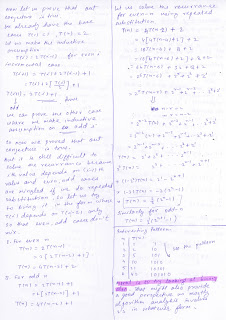### Discrete Maths, Lecture-6

These are my notes from 6th lecture of discrete mathematics course taught at Arsdigita university by Shai Simonson. Other posts relating to this are here.

In this lecture we're introduced to recursion and recurrence relations which arise in analysis of recursive algorithms, "repeated substitution"... the very basic technique of solving recurrence relations is covered with tower of hanoi puzzle as an example.

Here are my notes....## Monday, August 17, 2009

### Discrete Maths, ProblemSet#2

These are my solutions to 2nd problem set of discrete mathematics course taught at Arsdigita university by Shai Simonson. Other posts relating to this are here.

Here they are...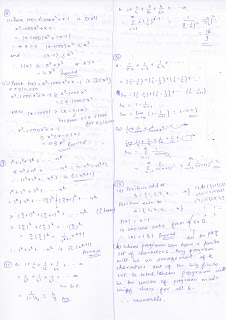### Discrete Maths, Lecture-5

These are my notes from 5th lecture of discrete mathematics course taught at Arsdigita university by Shai Simonson. Other posts relating to this are here.

In this lecture, Shai talks about functions and their types definitions i.e. one-one, onto and one-one onto... about comparison of order of growth of functions that involve exponents and log... and finally calculates sum of a series using the powerful concepts of manipulating known sums, describing the problem in appropriate notation(sigma notation).

Here are my notes...## Monday, August 10, 2009

### proof techniques

Proving things is an art, there is no mechanical way to do it. Like any other art one has to practice it to master it. One should get in the habit of asking why something is true/not true and then try to prove/disprove it.However, there are certain tricks/ways that can help shape the thinking when starting to think the proof.

For theorems of type, for All x: P(x)->Q(x)

Direct Proof:
We start with assuming that P(x) holds and then try to reach Q(x).

Proof by Contrapositive:
This proof technique uses the fact that contrapositive of p->q (which is -q->-p) has same truth value as p->q. So we can try to prove the contrapositive of original with direct proof technique.

Vacuous Proof:
If we can prove that P(x) is always false, then the statement p->q automatically becomes true.

Trivial Proof:
If we can prove that Q(x) is always true, no matter what.

For theorems of type, for all x: P(x)

Proof by Cases - Exhaustive Proof:
If x can only have a limited set of values, then we can apply P to *all* those values of x, if they all happens to be true(which has to be the case for theorem to be correct) then we have the proof.

Proof by Cases - Proof with limited Cases:
1. Some times, even though x can have a large(may be infinite) set of values but we can still use case techniue. For example let say P(x) is of the form of Q(sin(x)), then its enough to prove that P(x) holds for x E [0, PI], as sin(x) repeats itself for other values of x.
2. In cases something like x being real number, it is enough to show that P(x) holds for 3 cases which are.. x<0, x>0 and x=0

For theorems of type, there exists x | P(x)
It'll be enough to find just one value of x for which P(x) holds.

General
With this technique, we start with the assumption that negation of the theorem statement is true and reach a contradiction.

Try to find counter-example:
For example if we were to prove a theorem of type, for all x:P(x); if we can find one value of x for which P(x) is false then we have disproved the theorem.

Proof by Induction:
Here we prove a base case, Make an inductive assumption and prove the incremental case. Way to increment has to be general enough that all the possible cases are covered.

Reference - Section 1.6 and 1.7 of Discrete Mathematics & Its Applications 6th Edition by Kenneth H Rosen.

### Discrete Maths, Lecture-4

These are my notes from 4th lecture of discrete mathematics course taught at Arsdigita university by Shai Simonson. Other posts relating to this are here.

This lecture introduces the formalism to compare functions based on their order of growth. This is important for algorithms because actual running time of an algorithm is very sensitive to the implementation details, hardware its running on, load on the machine etc etc. So they can't be compared based on their actual running time but order of growth.

Here are my notes...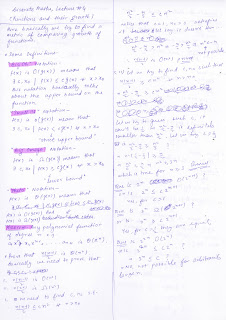## Sunday, August 9, 2009

### Programming In Scala: Chapter#6,7 notes

Ch6-
Fuctional objects = immutable objects, this chapter basically shows you how to write class for immutable Rational object. Many key concepts of writing classes in scala are covered.

In scala class, Two kind of constructors, primary and auxiliary. Its mandatory that an auxiliary constructor invokes anothe constructor.
Only primary constructor can invoke the constructor of superclass.

In scala class, Getters and setters are "generated" only for the field variables(unless they are declared private)

override keyword needs to be explicitly used when overriding a method of the superclass.

Though scala compiler allows you to use '\$' in identifier name, but it *MUST NOT* be used as its reserved for identifiers generated by scala compiler and there may be clashes.

Conventionally, identifiers for constants in java are like X_OFFSET, but in scall its recommended to use only first letter capital for constants and same identifier in scala should be XOffset.

A literal identifier trick can be used if you want to use a reserved word as identifier. A literal identifier is an identifier enclosed in back quotes.

Method overloading is supported that is same method name can be used for two different methods as long as there is some difference in method signature.

There is an implicit conversion trick that allows you to convert any type into any other type. Its very powerful but highly recommended not to be used and hence I'm not even mentioning here how its done :)

Ch7-
if, while, do-while, for, try, match - all return some value

Scala assignment always returns the unit value, ().

It recommends to use recursion rather than while loops to achieve the same effect.

for loop is swiss army knife in scala(seems inspired from loop macro in lisp), it can be used to do many different iteration tasks. Please see section 7.3 for its usage.

Scala's exceptions behave like same in java, thrown using throw keyword.Catching exception syntax is different a little bit and seems to less the code needed in handling multiple exceptions.

If a method throws an exception, its not mandatory for it to declare it using the throws as in java.

finally keyword is also available whose behavior is exactly as that in java.

"Match" is "switch" of java. However switch allows only matching of integers and enums whereas "Match" lets you select using arbitrary patterns.

## Saturday, August 8, 2009

### Programming In Scala: Chapter#4,5 notes

Ch4-
public is scala's default access level.

Method parameters in scala are val, they can't be reassigned.

In the absence of any returned statement, scala returns the last expression value computed by the method. The recommeded or the functional way is not to have explicit return statements and to definitely avoid multiple return statements. This philosophy encourages you to make small methods that just do one thing and not many.

Braces around the method definition are optional if there is only one expression inside the method body.

If you have a method whose return type is Unit, you can write it like following(removing the = sign) so that it looks like a procedure and clarifies the intention that its there for side effects only..

One thing to notice here is that whenever you write a function in procedural style(that is without equal sign), its infered return type is definitely going to be Unit no matter what the body contains.
A semicolon is *must* if you write multiple statements on a single line.

The rules of semicolon inference:
1. The line in question ends in a word that would not be legal as the end of a statement, such as a period or as an infix operator.
2. The next line begins with a word that cannot start with a statement.
3. The line ends while inside parentheses(...) or brackets [...], because these cannot contain multiple statements anyway.

Sigleton Objects: Scala classes can't have static fields or method, instead there can be singleton object whose definition looks exactly like scala classes with class replaced with keyword object. If there is a class with same name then singleton object is called companion object of that class, similarly that class is called companion class of this singleton object. Companion class/object can access private members of each other. A singleton object and the companion class *MUST* be defined in the same source file.

Ch5-
Other than the value types(corresponding to every primitive type in java) such as Byte, Short, Int, Long, Float, Double and Boolean, scala has a type called symbol which is like scheme symbols and literal representation is same as scheme symbols, they start with single quote e.f.'symbol

Symbols are interned, if you write the same symbol twice, they both refer to the same symbol object.

unary_x, where x = +/-/!/~ can be used in prefix notation that is 2.unary_- is same as -2.

If a method takes no arguments, it can be written in postfix notation, that is s.toStrin() can be written as "s toString".

A == B in scala first checks to see if A is null, if its not then it checks
A.equals(B). In java '==' compares referential equality which here is done with method eq(this only applies to the objects which can be directly mapped into java objects)

Scala doesn't have operators, so how does 2+2*7 return 16. The answer is that precedence is based on the first letter of the method name and method starting with * have higher precedence than methods starting with +.

The associativity(when there are multiple methods with same precendence) is determined by the last character of the method name.

For every basic scala type, there is a rich wrapper that provides more utility methods on it.

### p->q <=> p only if q <=> q unless -p

Note: '-p' represents NOT p.

As an student whenever I learned above, I always used to be confused if the three were equivalent. Here is how I can remember them now.

Let say p = I'll eat banana.
q = Its not evening.

p only if q
The sentence, "I'll eat banana ONLY if Its not evening." is equivalent to "If I eat banana that means its not evening" which is p->q

q unless -p
The sentence, "Its not evening unless I'm not eating banana." is equivalent to "Its not evening if I'm eating banana" which is "If I'm eating banana then It's not evening" which p->q

One more important thing I learned is that mathematical logic statements have nothing to do with same in natural languages such as english, we draw this parallel just to remember them better. Natural language statements are often ambiguous but logic statements always have a precise meaning.

## Wednesday, August 5, 2009

### Discrete Maths, Lecture-3

These are my notes from 3rd lecture of discrete mathematics course taught at Arsdigita university by Shai Simonson. Other posts relating to this are here.

This lecture introduces the Set theory and how it relates to Logic. It shows how most of the things in Set theory can be proved using the laws relating to AND, OR that we learned in logic. Shai also discusses two nice tricks to do counting that has something to do with sets.

Here are my notes...## Monday, August 3, 2009

### Discrete Maths, ProblemSet#1

These are my solutions to 1st problem set of discrete mathematics course taught at Arsdigita university by Shai Simonson. Other posts relating to this are here.

Scheme Programs are typed here, others are scanned...
P3.a-
`(define (find-goals-misses score)  (define (aux goals near-misses)    (let ((tmp (+ (* 11 goals) (* 7 near-misses))))      (cond       ((> score tmp) (aux (+ goals 1) near-misses))       ((< score tmp) (aux goals (- near-misses 1)))       (else  `((goals ,goals) (near-misses ,near-misses))))))  (aux 0 (quotient score 7)))`

P8-
`;following code is copied from sicp;changed a lit bit to make it run on plt-r5rs(define-syntax cons-stream  (syntax-rules ()    ((cons-stream a b)     (cons a (delay b)))))(define (stream-car stream) (car stream))(define (stream-cdr stream) (force (cdr stream)))(define stream-null? null?)(define the-empty-stream '())(define (stream-filter pred stream)  (cond ((stream-null? stream) the-empty-stream)        ((pred (stream-car stream))         (cons-stream (stream-car stream)                      (stream-filter pred                                     (stream-cdr stream))))        (else (stream-filter pred (stream-cdr stream)))))(define (stream-ref s n)  (if (= n 0)      (stream-car s)      (stream-ref (stream-cdr s) (- n 1))))(define (integers-starting-from n)  (cons-stream n (integers-starting-from (+ n 1))))(define primes  (cons-stream   2   (stream-filter prime? (integers-starting-from 3))))(define (square x) (* x x))(define (divisible? x y) (= (remainder x y) 0))(define (prime? n)  (define (iter ps)    (cond ((> (square (stream-car ps)) n) #t)          ((divisible? n (stream-car ps)) #f)          (else (iter (stream-cdr ps)))))  (iter primes));solution to the problem(define (multiply-n-primes n)  (define (iter ps n a)    (if (= n 0) a (iter (stream-cdr ps) (- n 1) (* a (stream-car ps)))))  (iter primes n 1))(define (find-p8-n)  (define (iter i)    (let ((tmp (+ 1 (multiply-n-primes i))))    (if (prime? tmp) (iter (+ i 1)) tmp)))  (iter 1));Ans: 30031`
Here are the other solutions...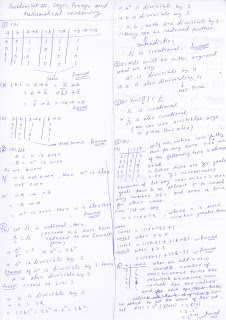### Programming In Scala: Chapter#2,3 notes

I did a quick reading of above mentioned chapters from Programming in Scala by Martin Odersky and noted a few key things...

A scala compiler does not infer function parameter types, it does so only for the function return type.

If function is recursive, you must specify its return type.

If function body has only a single expression then you can optionally leave out the curly braces.

"Unit" type is returned from the functions that don't do anything useful, its like java void.

If a function literal consists of only one expression that takes the argument then we may skip writing the argument explicitly. Let me give an example..
`array.forEach(arg => println(arg))is equivalent toarray.forEach(println)`
There are two kinds of variables in scala, var and val. val are final and can't be reassigned. Although the variables defined with val are final. but the object, they are refering to, might very well be mutable.

If a method takes only one parameter then you can call it without using the parenthesis. In reality there are no operators in scala(as in scheme:)) and the op looking variables are actually functions taking advantage of the mentioned fact. For example, "1 + 2" is actually "1.+(2)"
If a method is used in operator notation like a op b, then it means a.op(b) except in the case where method name ends with colon and then "a op: b" mean b.op:(a)

If you say greet("hi") on a variable, it automatically gets converted to greet.apply("hi") that is why array elements can be accessed with array(index) syntax. Similarly greet("hi")=value is transformed into greet.update("hi",value).

Pretty much for every data structure, scala provides both, mutable and immutable implementations.

Tuples can be used for multiple value return from a function.

semicolons are mostly optional.

Scala supports functional as well as imperative programming but encourages to use functional unless its justifiable to use the other one and hence prefer val over var, immutable object over mutable object and functions with no side effects over functions with side effects.

## Sunday, August 2, 2009

### Discrete Maths, Lecture#2

These are my notes from 2nd lecture of discrete mathematics course taught at Arsdigita university by Shai Simonson. Other posts relating to this are here.

This lecture starts with Shai describing how mathematicians "reach" the proof. They start with trying to convince the other person about the new idea they've come up with and asking questions like.. do you get what I mean?... do you believe that its true? And that is how proofs begin... does it seem reasonable?.. does it seem plausible?.. do you believe in it? So basically you think and think and think, you get the idea, you check the idea, if found a hole you plug the the hole.. then check again..iterate this process and once all the holes are filled.. then you write the idea in english or in rigorous mathematical notation.

However when you need to prove something, first you should try to convince yourself that the idea you're trying to prove is indeed true by way of taking particular examples and see its true. Once you've convinced yourself and have a better understanding of the idea then you can think about the proof better.

On a high level this lecture answers following questions..
How to build electric circuits using truth table?
How to do arithmatic(addition in particular) using circuits and logic?
High level notion of NP and NP-complete problems?
Using reduction to prove if a problem is NP-complete or not?

Here are my notes...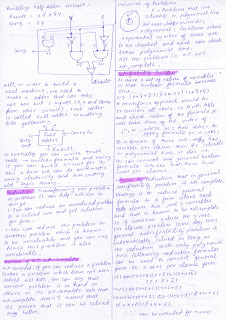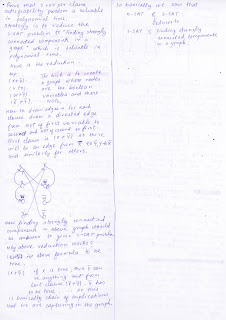## Saturday, August 1, 2009

### Installing Fedora11 from Hard-disk

I have had Fedora-9 installed since long and wanted to move to Fedora-11. I also have windows xp already installed, Here is how I installed Fedora-11 from the hard disk itslef...

• Booted the machine in windows, downloaded the fedora11 dvd iso in one partition(d:\, its FAT32).

• Extracted images/install.img from fedora archive into d:\images\

• Extracted vmlinuz, initrd.img from isolinux directory in the fedora archive into c:\boot\ from the above iso.

• Edited c:\boot\grub\menu.lst, and appended following..
title my fedora-11 install
kernel (hd0,0)/boot/vmlinuz
initrd (hd0,0)/boot/initrd.img

• Extracted file grldr from grub4dos-0.4.1.zip to c:\.

• Edit boot.ini and ..append c:\grldr="Start Linux" at the end with a newline.

• Reboot the machine, select "my fedora-11 install" , on prompt for source of installation select Hard-disk, then select the partition where you saved the fedora iso and then the anaconda installer launches. I like to keep SElinux disabled.